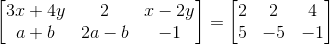Find x, y, a and b if

Asked by Aaryan | 1 year ago |  138

##### Solution :-

GivenGiven that two matrices are equal.

We know that if two matrices are equal then the elements of each matrices are also equal.

Therefore by equating them we get,

3x + 4y = 2 …… (1)

x – 2y = 4 …… (2)

a + b = 5 …… (3)

2a – b = – 5 …… (4)

Multiplying equation (2) by 2 and adding to equation (1), we get

3x + 4y + 2x – 4y = 2 + 8

⇒ 5x = 10

⇒ x = 2

Now, substituting the value of x in equation (1)

3 × 2 + 4y = 2

⇒ 6 + 4y = 2

⇒ 4y = 2 – 6

⇒ 4y = – 4

⇒ y = – 1

Now by adding equation (3) and (4)

a + b + 2a – b = 5 + (– 5)

⇒ 3a = 5 – 5 = 0

⇒ a = 0

Now, again by substituting the value of a in equation (3), we get

0 + b = 5

⇒ b = 5

∴ a = 0, b = 5, x = 2 and y = – 1

Answered by Aaryan | 1 year ago

### Related Questions

#### Find the values of a, b, c and d from the equations

Find the values of a, b, c and d from the equations:

#### Find x, y, a and b if

Find x, y, a and b if

#### Construct a 4 × 3 matrix A = [ai j] whose elements ai j are given by ai j = i

Construct a 4 × 3 matrix A = [ai j] whose elements ai j are given by ai j = i

Construct a 4 × 3 matrix A = [ai j] whose elements ai j are given by $$a_{i j} = \dfrac{(i – j)}{(i + j)}$$
Construct a 4 × 3 matrix A = [ai j] whose elements ai j are given by ai j = 2i + $$\dfrac{i}{j}$$